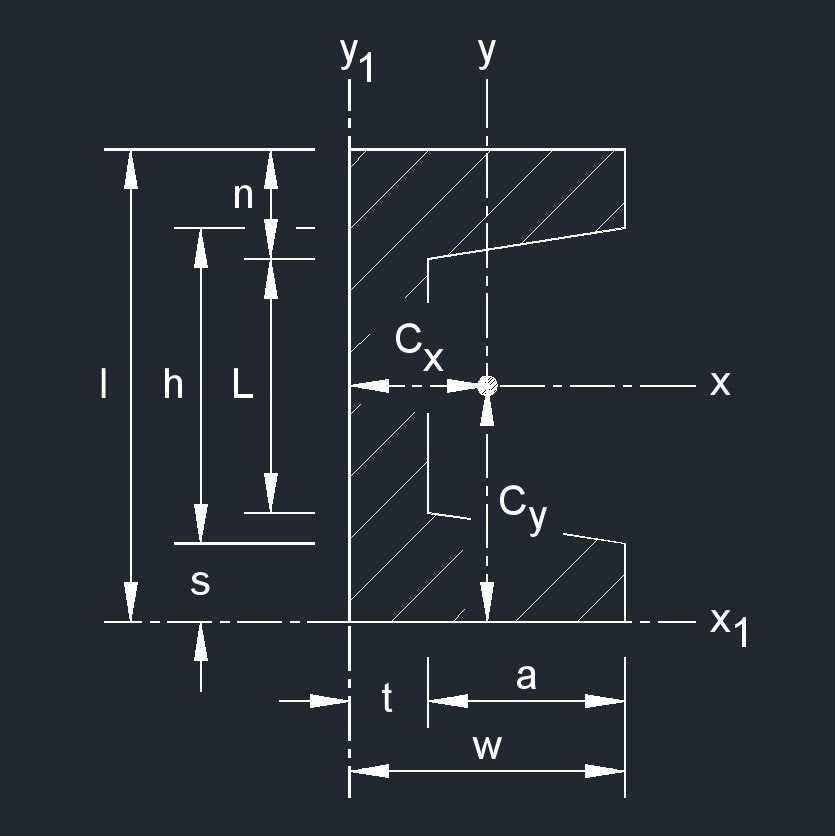# Tapered Channel

Written by Jerry Ratzlaff on . Posted in Structural Engineering

•A tapered channel is a structural shape used in construction.

## area of a Tapered Channel formula

 $$\large{ A = l\;t + a \; \left( s + n \right) }$$

### Where:

$$\large{ A }$$ = area

$$\large{ l }$$ = height

$$\large{ n }$$ = thickness

$$\large{ s }$$ = thickness

$$\large{ t }$$ = thickness

$$\large{ a }$$ = width

## Distance from Centroid of a Tapered Channel formulas

 $$\large{ C_x = \frac{1}{3} \; \left[ w^2 \;s + \frac{h\;t^2}{2} \;-\; \frac{\frac{h \;-\; L}{2\;\left(w \;-\; t \right) } }{3} \; \left( w + 2\;t \right) \left( w - t \right)^2 \right] }$$ $$\large{ C_y = \frac{l}{2} }$$

### Where:

$$\large{ C }$$ = distance from centroid

$$\large{ h }$$ = height

$$\large{ l }$$ = thickness

$$\large{ L }$$ = thickness

$$\large{ s }$$ = thickness

$$\large{ t }$$ = thickness

$$\large{ w }$$ = width

## Elastic Section Modulus of a Tapered Channel formulas

 $$\large{ S_x = \frac{ I_x }{ C_y } }$$ $$\large{ S_y = \frac{ I_y }{ C_x } }$$

### Where:

$$\large{ S }$$ = elastic section modulus

$$\large{ C }$$ = distance from centroid

$$\large{ I }$$ = moment of inertia

## Perimeter of a Tapered Channel formula

 $$\large{ P = 2\;a^2 + 2\;w + 2\;h - 2\;L^2 + L + 2\;s }$$

### Where:

$$\large{ P }$$ = perimeter

$$\large{ h }$$ = height

$$\large{ L }$$ = height

$$\large{ s }$$ = thickness

$$\large{ a }$$ = width

$$\large{ w }$$ = width

## Polar Moment of Inertia of a Tapered Channel formulas

 $$\large{ J_z = I_x + I_y }$$ $$\large{ J_{z1} = I_{x1} + I_{y1} }$$

### Where:

$$\large{ J }$$ = torsional constant

$$\large{ I }$$ = moment of inertia

## Radius of Gyration of a Tapered Channel formulas

 $$\large{ k_x = \sqrt{ \frac{ \frac{1}{12} \; \left[ w\;l^3 \;+\; \frac{1}{8\;\frac{h \;-\; L}{2\;\left(w \;-\; t \right)}} \; \left( h^4 \;-\; L^4 \right) \right] }{ lt \;+\; a \;\left( s \;+\; n \right) } } }$$ $$\large{ k_y = \sqrt{ \frac{ \frac{1}{3} \; \left[ 2\;s\;w^3\; L\;t^3 \;+\; \frac{\frac{h \;-\; L}{2\;\left(w \;-\; t \right)}}{2} \; \left( w^4 \;-\; t^4 \right) \right] \;-\; A \; \left( w \;-\; y \right)^2 }{ l\;t \;+\; a\; \left( s \;+\; n \right) } } }$$ $$\large{ k_z = \sqrt{ k_{x}{^2} + k_{y}{^2} } }$$ $$\large{ k_{x1} = \sqrt{ \frac{ I_{x1} }{A} } }$$ $$\large{ k_{y1} = \sqrt{ \frac{ I_{y1} }{A} } }$$ $$\large{ k_{z1} = \sqrt{ k_{x1}{^2} + k_{y1}{^2} } }$$

### Where:

$$\large{ k }$$ = radius of gyration

$$\large{ h }$$ = height

$$\large{ l }$$ = height

$$\large{ L }$$ = height

$$\large{ s }$$ = thickness

$$\large{ t }$$ = thickness

$$\large{ a }$$ = width

$$\large{ w }$$ = width

## Second Moment of Area of a Tapered Channel formulas

 $$\large{ I_x = \frac{1}{12} \; \left[ w\;l^3 + \frac{1}{8\;\frac{h \;-\; L}{2\;\left(w \;-\; t \right) }} \; \left( h^4 \;-\; L^4 \right) \right] }$$ $$\large{ I_y = \frac{1}{3} \; \left[ 2\;s\;w^3 + L\;t^3 + \frac{\frac{h \;-\; L}{2\;\left(w \;-\; t \right) }}{2} \; \left( w^4 \;-\; t^4 \right) \right] \;-\; A \left( w \;-\; y \right)^2 }$$ $$\large{ I_{x1} = l_x + A\;C_y }$$ $$\large{ I_{y1} = l_y + A\;C_x }$$

### Where:

$$\large{ I }$$ = moment of inertia

$$\large{ A }$$ = area

$$\large{ C }$$ = distance from centroid

$$\large{ h }$$ = height

$$\large{ l }$$ = height

$$\large{ L }$$ = height

$$\large{ s }$$ = thickness

$$\large{ t }$$ = thickness

$$\large{ w }$$ = width

## Torsional Constant of a Tapered Channel formula

 $$\large{ J = \frac{ 2 \; \left( w \;-\; \frac {t}{2} \right) \; n^3 \; \left( l \;-\; n \right) \; t^3 }{3} }$$

### Where:

$$\large{ J }$$ = torsional constant

$$\large{ l }$$ = height

$$\large{ n }$$ = thickness

$$\large{ s }$$ = thickness

$$\large{ t }$$ = thickness

$$\large{ w }$$ = width